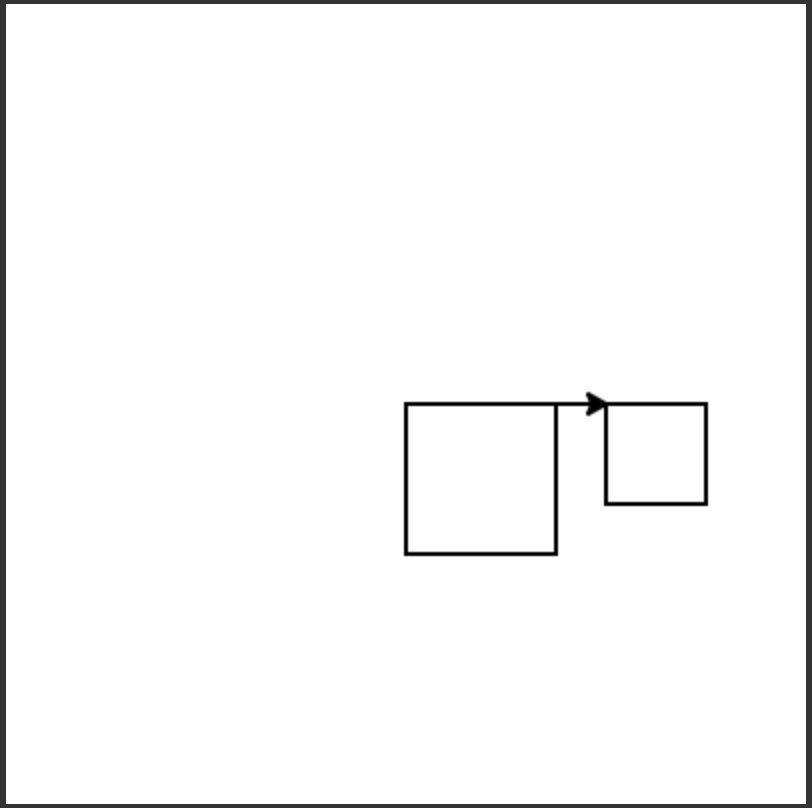# 6.4. Naming Input¶

What if we want to draw a different size square, like one with a side length of 50? We could change each of the calls to the forward procedure as shown below.

But, this means we have to change each of the four forward statements and we could make a mistake and not set all of them to the same number. Is there a better way? What if we create a variable size and set its value to the amount to move forward?

Now the program is easier to change since we only have one line to change size = 50 to draw another size square. But, we still have to change the program in order to draw a different size square. Is there a better way?

We can add an additional input to the function that specifies the size of the square. Just separate the names for the inputs with a comma: (turtle,size) as shown below and be sure to specify the actual size when you call the procedure square(malik, 100) or square(malik, 50).

The inputs that are specified in a function or procedure definition are also called parameters or formal parameters. So turtle and size are both parameters (formal parameters) in the square procedure. Notice that when we call square we have to specify the actual values for the inputs. The actual values passed into the function as inputs are called the arguments or actual parameters. In the call square(malik, 50) both malik and 50 are arguments (actual parameters) to the square procedure.

The following code assumes that a procedure square has been defined that takes a size. The code should create a turtle and then use it to draw a square, move forward, and draw a second square as shown below, but the lines are mixed up.Note

Discuss topics in this section with classmates.